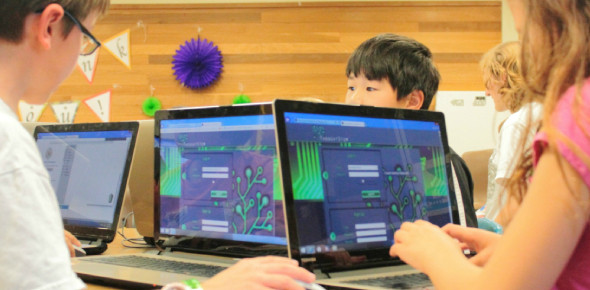# Blind Coding C Programming Quiz!

50 Questions | Attempts: 459Settings.

• 1.
Point out the correct statements are correct about the program below? #include int main() {     char ch;     while(x=0;x<=255;x++)         printf("ASCII value of %d character %c\n", x, x);     return 0; }
• A.

The code generates an infinite loop

• B.

The code prints all ASCII values and its characters

• C.

Error: x undeclared identifier

• D.

Error: while statement missing

• 2.
Which of the following is the correct usage of conditional operators used in C?
• A.

A. a>b ? c=30 : c=40;

• B.

A>b ? c=30;

• C.

Max = a>b ? a>c?a:c:b>c?b:c

• D.

Return (a>b)?(a:b)

• 3.
If a function contains two return statements successively, the compiler will generate warnings. Yes/No?
• A.

Yes

• B.

No

• 4.
What will be the output of the program ? #include int main() {     float arr[] = {12.4, 2.3, 4.5, 6.7};     printf("%d\n", sizeof(arr)/sizeof(arr));     return 0; }
• A.

5

• B.

4

• C.

6

• D.

7

• 5.
Is there any difference int the following declarations? int fun(int arr[]); int fun(int arr);
• A.

Yes

• B.

No

• 6.
Does there exist any way to make the command-line arguments available to other functions without passing them as arguments to the function?
• A.

Yes

• B.

No

• 7.
What function should be used to free the memory allocated by calloc()?
• A.

Dealloc();

• B.

Malloc(variable_name, 0)

• C.

Free();

• D.

Memalloc(variable_name, 0)

• 8.
What do the following declaration signify? void *cmp();
• A.

Cmp is a pointer to an void type

• B.

Cmp is a void type pointer variable.

• C.

Cmp is a function that return a void pointer.

• D.

Cmp function returns nothing.

• 9.
What will be the output of the program? #include int main() {     char huge *near *far *ptr1;     char near *far *huge *ptr2;     char far *huge *near *ptr3;     printf("%d, %d, %d\n", sizeof(**ptr1), sizeof(ptr2), sizeof(*ptr3));     return 0; }
• A.

4, 4, 4

• B.

2, 2, 2

• C.

2, 8, 4

• D.

2, 4, 8

• 10.
We can modify the pointers "source" as well as "target".
• A.

True

• B.

False

• 11.
Will the program outputs "IndiaBIX.com"?  #includeint main(){    char str1[] = "IndiaBIX.com";    char str2;    strncpy(str2, str1, 8);    printf("%s", str2);    return 0;}
• A.

Yes

• B.

No

• 12.
Which of the following cannot be checked in a switch-case statement?
• A.

Character

• B.

Integer

• C.

Float

• D.

Enum

• 13.
Which of the following statements are correct about the program? #include int main() {     int x = 30, y = 40;     if(x == y)         printf("x is equal to y\n");       else if(x > y)         printf("x is greater than y\n");       else if(x < y)         printf("x is less than y\n")     return 0; }
• A.

Error: Statement missing

• B.

Error: Expression syntax

• C.

Error: Lvalue required

• D.

Error: Rvalue required

• 14.
Are the following two statement same?                      1.         a <= 20 ? (b = 30): (c = 30);                      2.         (a <=20) ? b : (c = 30);
• A.

Yes

• B.

No

• 15.
What will be the output of the program ?#include int main(){    char *str;    str = "%d\n";    str++;    str++;    printf(str-2, 300);    return 0;}
• A.

No output

• B.

30

• C.

3

• D.

300

• 16.
The library function used to reverse a string is ____
• A.

Strstr()

• B.

Strrev()

• C.

Revstr()

• D.

Strreverse()

• 17.
Point out the error in the program? struct emp {     int ecode;     struct emp *e; };
• A.

Error: in structure declaration

• B.

• C.

No Error

• D.

None of above

• 18.
In Turbo C/C++ under DOS if we want that any wild card characters in the command-line arguments should be appropriately expanded, are we required to make any special provision?
• A.

Yes

• B.

No

• 19.
Bitwise | can be used to multiply a number by powers of 2.
• A.

Yes

• B.

No

• 20.
What does the following declaration signify? int *f();
• A.

F is a pointer variable of function type.

• B.

F is a function returning pointer to an int.

• C.

F is a function pointer.

• D.

F is a simple declaration of pointer variable.

• 21.
Which of the following is the correct order of evaluation for the below expression? z = x + y * z / 4 % 2 - 1
• A.

* / % + - =

• B.

= * / % + -

• C.

/ * % - + =

• D.

* % / - + =

• 22.
Will the printf() statement print the same values for any values of a?#includeint main(){    float a;    scanf("%f", &a);    printf("%f\n", a+a+a);    printf("%f\n", 3*a);    return 0;}
• A.

Yes

• B.

No

• 23.
What will be the output of the program if the array begins 1200 in memory?#include int main(){    int arr[]={2, 3, 4, 1, 6};    printf("%u, %u, %u\n", arr, &arr, &arr);    return 0;}
• A.

1200, 1202, 1204

• B.

1200, 1200, 1200

• C.

1200, 1204, 1208

• D.

1200, 1202, 1200

• 24.
What will be the output of the program? #include   int main() {     printf(5+"IndiaBIX\n");     return 0; }
• A.

Error

• B.

IndiaBIX

• C.

BIX

• D.

None of above

• 25.
Point out the error in the program? struct emp {     int ecode;     struct emp e; };
• A.

Error: in structure declaration

• B.

• C.

No Error

• D.

None of above

## Related TopicsBack to top
×

Wait!
Here's an interesting quiz for you.